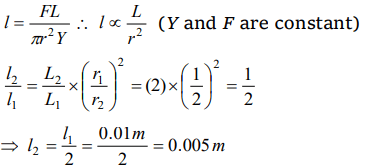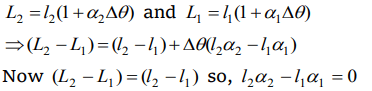## Mechanical Properties of Solids Questions and Answers Part-8

1. To keep constant time, watches are fitted with balance wheel made of
a) Invar
b) Stainless steel
c) Tungsten
d) Platinum

Explanation: Because dimension of invar does not varies with temperature

2. A wire is stretched by 0.01 m by a certain force F. Another wire of same material whose diameter and length are double to the original wire is stretched by the same force. Then its elongation will be
a) 0.005 m
b) 0.01 m
c) 0.02 m
d) 0.002 m

Explanation:3. The possible value of Poisson's ratio is
a) 1
b) 0.9
c) 0.8
d) 0.4

Explanation: Poisson’s ratio varies between – 1 and 0.5

4. The coefficient of linear expansion of brass and steel are $\alpha_{1}$ and $\alpha_{2}$ . If we take a brass rod of length $l_{1}$ and steel rod of length $l_{2}$ at 0°C, their difference in length$\left(l_{2}-l_{1}\right)$  will remain the same at a temperature if
a) $\alpha_{1}l_{2}=\alpha_{2}l_{1}$
b) $\alpha_{1}l_2^2=\alpha_{2}l_1^2$
c) $\alpha_1^2l_{1}=\alpha_2^2l_{2}$
d) $\alpha_{1}l_{1}=\alpha_{2}l_{2}$

Explanation:5. A rod is fixed between two points at 20°C. The coefficient of linear expansion of material of rod is $1.1\times 10^{-5}\diagup^{\circ}C$    and Young's modulus is $1.2\times 10^{11}N\diagup m$   . Find the stress developed in the rod if temperature of rod becomes 10°C
a) $1.32\times 10^{7}N\diagup m^{2}$
b) $1.10\times 10^{15}N\diagup m^{2}$
c) $1.32\times 10^{8}N\diagup m^{2}$
d) $1.10\times 10^{6}N\diagup m^{2}$

Explanation:6. The extension of a wire by the application of load is 3 mm. The extension in a wire of the same material and length but half the radius by the same load is
a) 12 mm
b) 0.75 mm
c) 15 mm
d) 6 mm

Explanation:7. A rubber pipe of density $1.5\times 10^{3}N\diagup m^{2}$   and Young's modulus $5\times 10^{6}N\diagup m^{2}$   is suspended from the roof. The length of the pipe is 8 m. What will be the change in length due to its own weight
a) 9.6 m
b) $9.6 \times 10^{3}m$
c) $19.2 \times 10^{-2}m$
d) $9.6 \times 10^{-2}m$

Explanation:8. In which case there is maximum extension in the wire, if same force is applied on each wire
a) L = 500 cm, d = 0.05 mm
b) L = 200 cm, d = 0.02 mm
c) L = 300 cm, d = 0.03 mm
d) L = 400 cm, d = 0.01 mm

Explanation:9.If a spring is extended to length l, then according to Hook's law
a) $F=kl$
b) $F=\frac{k}{l}$
c) $F=k^{2}l$
d) $F=\frac{k^{2}}{l}$

Explanation: $F=kl$